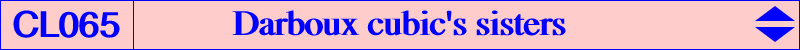This page is a sequel to the FG paper How pivotal cubics intersect the circumcircle. See also the related Table 58. Let P be a finite point and let K(P) = pK(X6, P) be the isogonal pivotal cubic with pivot P, meeting the circumcircle (O) of ABC at three points Q1, Q2, Q3. These are not always all real nor distinct and the orthocenter of Q1Q2Q3 is P. K(P) is then a circumcubic in Q1Q2Q3 and we denote by DK(P) its transform under the isogonal conjugation F with respect to Q1Q2Q3. DK(P) is obviously also a circumcubic in Q1Q2Q3 and it must pass through the circumcenter O of ABC. DK(P) is actually a central cubic with center O and with three real asymptotes which are those of the Darboux cubic K004, namely the perpendicular bisectors of ABC. Their infinite points are the F images of A, B, C. DK(P) is called the P-sister of K004 and DK(X20) is K004 itself. It follows that DK(P) and K004 meet at three double points at infinity and three remaining finite collinear points which are O and P1, P2 symmetric in O. The line passing through these points also contains gcP, the isogonal conjugate (in ABC) of the complement cP of P. O is a point of inflexion and the tangent at O passes through the perspector of the tangential triangle (anticevian triangle of X6) and the circumcevian triangle of gP. This is also known as the first Saragossa point of gP. The orthic line of DK(P) is the Euler line of ABC hence, for any P, the polar conic of each point on the Euler line is a rectangular hyperbola. That of O splits into the tangent at O and the line at infinity. These hyperbolas are homothetic to the Kiepert hyperbola (hence passing through X3413, X3414) and their centers lie on a line passing through O. *** The following table gives a selection of these cubics (with the collaboration of Peter Moses).P K(P) DK(P) Xi on DK(P) for i = Q1Q2Q3 notes 2 K002 K758 2, 3, 154, 165, 376, 3576, 10606 Thomson triangle (1) 3 K003 3 / vertices of the CircumTangential triangle CircumNormal triangle (1), (3) 4 K006 3, 4, 20, 1158, 6261, 9732, 9733 (1) 6 K102 3, 182, 3098 Grebe triangle 7 1, 3, 9, 40, 2550, 5732 20 K004 K004 see the page antipodal triangle (1) 69 K169 3, 4, 6, 20, 990, 1350, 1766 99 K035 3, 98, 99, 1379, 1380 X(99), X(1379), X(1380) (2) 100 3, 100, 104, 1381, 1382 X(100), X(1381), X(1382) (2) 110 K316 3, 74, 110, 1113, 1114 X(110), X(1113), X(1114) (2) 144 1, 3, 40, 390, 2951, 11372 193 3, 4, 20, 1351, 1721, 12652, 12717 329 3, 57, 84, 497, 1490, 6282, 10860 385 K128 3, 98, 99, 2080, 5999, 11676 476 K130 3, 74, 110, 476, 477 X(74), X(110), X(476) (2) 3146 3, 64, 1498, 3146, 3529, 7971, 7992 (1) 3580 3, 74, 110, 858, 10295, 10564 5999 K422 3, 98, 99, 2080, 5999, 11676 (1) 6840 3, 1768, 5535, 5538, 6326, 6840 (1)Notes : • DK(P) passes through P if and only if (1) : either P lies on the Euler line in which case K(P) and DK(P) meet at O, P, Q1, Q2, Q3 and four other points on K800. These points lie on two perpendicular lines secant at cP. (2) : or P lies on the circumcircle (O). Q1 = P and Q2, Q3 lie on the parallel at O to the Simson line of P. DK(P) is bitangent to (O) at these two points. See Table 17. • (3) : DK(X3) is a member of the pencil of cubics generated by K735 and the union of the Euler line and (O). All these cubics pass through the vertices of the CircumTangential and CircumNormal triangles, O which is an inflexion point with tangent the Euler line, and they meet the line at infinity at the same points as a pK(X6, S) where S is a point on the Euler line. Obviously, S = X(20) for DK(X3), S = X(2) for K735. See Table 25.